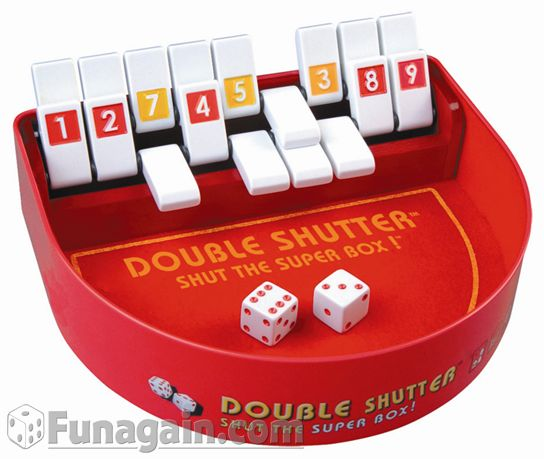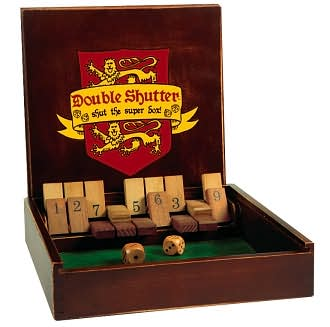# (Double Shutter) Shut The Box Winning Strategy

Home --> Programming Projects --> Shut The Box (Winning Strategy)
For family fun my dad got the game "Double Shutter: Shut The Super Box" (see pictures below). I thought I would be a smarty-pants and compute an optimal strategy for the game using a computer so that is what I did! This game is a variant of the traditional "Shut The Box" game, where this version uses two rows of tiles (9 tiles in a row) instead of one. Durango Bill already performed an analysis of the optimal strategy for various modifications of the original game, but it looks like the double-shutter variation is new teritory! For a description of the original game, see Wikipedia.Here are the rules of the game: Each turn the player rolls two 6 sided die and the player must "shut" enough standing tiles so that the sum of the values of the tiles shut equals the sum of the values on the two die just rolled. The goal of the game is to get a low score. If the player cannot do this, the game is over and his score is the sum of the values of the tiles in the back row plus twice the values of the tiles in the front row. As an exception, a player may choose to instead roll one die if the sum of the values of the exposed tiles is less than or equal to 6 (a player would be crazy not to make this choice if he is able to). Consider the above picture to the left. If the player rolls 10, he can shut the left-most 1 tile and then shut the 9 tile behind it and this counts as a valid move.

Suppose that the object of playing the game is to get the least expected value. Here is how to compute a winning strategy for the game: Let a board be defined as a valid configuration of tiles in a box as depicted by the pictures above. If b is a board, let rank(b) denote the number of tiles standing in b. Let adj(b,r) denote the set of all boards that could be reached (in one move) from b given that r has been roled. Let prob(r) denote the probability or rolling r (let us ignore the issue of rolling two versus one die in this discussion). Let E(b) denote the expected score of the game given that the board is currently b. Let score(b) denote twice the sum of the values on the standing tiles in the front row plus the sum of the values on the standing tiles in the back row. We have the recursive relationship:

E(b) = sum (from r = 2 to 12) of prob(r) * A(b,r)
A(b,r) = min { E(b') | b' in adj(b) } if adj(b) is non-empty
A(b,r) = score(b) otherwise

The key is that if b' in adj(b) then rank(b') < rank(b). This means that E(b) can be computed using dynamic programming: computing the expected values for all boards of rank R given the expected values of all boards of rank < R. The only anoying part is enumerating all boards of rank R and determining adj(b) for a given b. The (Haskell) source code used to brute force this program can be found here and the computed optimal strategy (board expected values) can be found here. Notice how a table of computed expected values along with a way to compute adj is all that is needed to play the game optimally. It turns out that the expected value for the game (playing optimally) is "19.427".

If instead the point of the game is not to get the least expected value but to get a score of 0 with the highest probability, then we would want to use a different (all or nothing) strategy! Indeed, all we do is change the third line in the above recursive relationship to the following:

A(b,r) = (0 if score(b) == 0 and 1 otherwise) otherwise

In this case, the expected value is just the probability of not getting a score of 0 when playing optimally. The computed board expected values for this can be found here here. It turns out that the probability for not getting a score of 0 (playing optimally) is "0.923". More generally, here is a graph showing the probability p(n) of not getting a score <= n given an optimal strategy for trying to do so:Exact values for p(n) for n = 1,...,20 can be found here. It is a bit anoying that the optimal strategy does not make winning much more likley. A comparison of the optimal strategy with the strategy of making moves randomly would be interesting for future work.